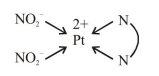# The number of isomers possible for

Question:

The number of isomers possible for $\left[\mathrm{Pt}(\mathrm{en})\left(\mathrm{NO}_{2}\right)_{2}\right]$ is :

1. 3

2. 2

3. 1

4. 4

Correct Option: 1

Solution:

$\left[\mathrm{Pt}(\right.$ en $\left.)\left(\mathrm{NO}_{2}\right)_{2}\right] \Rightarrow$ does not show G.I. as well as optical isomerism.This complex will have three linkage isomers as follows :-

[Pt (en) $\left.\left(\mathrm{NO}_{2}\right) 2\right] \mathrm{I}$

[Pt (en) $\left.\left(\mathrm{NO}_{2}\right)(\mathrm{ONO})\right] \mathrm{II}$

$\left[\mathrm{Pt}(\mathrm{en})(\mathrm{ONO})_{2}\right] \mathrm{III}$